## Finding dominant colors in an image

Here's a simple task - given an image find the dominant colors in the image. I'll walk you through a lesser known technique that does not use kmeans.

## Eigenvectors

Series: Finding dominant colors in an image:

Ever wondered how that android notification light seems to know the color of the application? If it is a notification from Facebook, it is blue in color. If it is a gmail notification, it shows up red. And of course, snapchat is yellow. How does Android figure out which color to use?

These are trivial examples. What you'll learn in this tutorial is much more versatile and will help you get started with better color based segmentation. The best part about this technique is that it is deterministic.

## Can we simply use kmeans?

Definitely. In fact, the code for it is much more straight forward given that most machine learning packages these days have a kmeans method in them. However, it may not be deterministic (you'll get different results each time). This is because the initialization of kmeans greatly determines if you'll get good clusters or not. I cover this in a previous tutorial on kmeans clustering.

To overcome this, a standard practice is to use either known initializations or run kmeans several times and pick the best clustering result. In the first case, you're not guaranteed to get the best colors. In the second case, you're increasing your runtime.

Can we do better? That's what this tutorial covers.

## Color Quantization

To solve this problem, we'll look at an approach in color quantization. The idea is that you have an image with millions of colors - but the hardware is limited and can only display a certain number of colors (think old LCD displays with only 256 colors).

If we dial down the number of available colors (to something like 4-5), we get the dominant colors in the image. These dominant colors are mathematically the best possible colors to display the image with the least amount of error.

Let's jump into some theory and then we'll implement it with OpenCV in the next part.Notice how the approximation of the image improves as the number of palette colors increases (from 1 to 6). By the time we're at 6 colors, the approximation is starting to look pretty good.

## Hierarchical quantization

Let's start with an image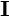where each pixel has an RGB value associated with it. We start off by assuming that the entire image is made up of a single color. Then we successively split this node to get the required number of colors.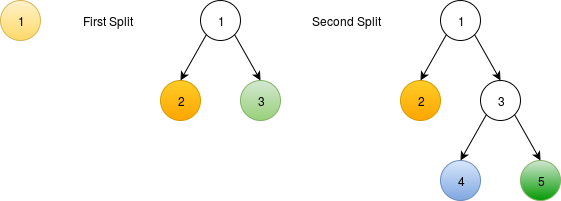Splitting dominant colors using a binary tree. The leaf nodes in the tree are the dominant colors. To match the batman example, we'd have to split a total of 5 times to produce 6 dominant colors.

Each node is associated with a "class". When you start out this process, each pixel in the image is assigned the class 1. After performing the first split, each pixel either belongs to class 2 or class 3. Similarly, after the second split, each pixel belongs to either class 2, 4 or 5.How quantization works. The first image is the original and each subsequent step is how each pixel is assigned a different class based on its pixel color.

In the above image, the blue colored image is where each pixel is assigned the same class. This reprepresents the very first tree. Then we split this class into two (disjoint) sets - represented here by red and green. Then the green is split into two further nodes - yellow and cyan.

Notice how each of these classes seem to follow a certain color - yellow seems to follow grays in the original image and cyan follows the blacks.

The yellow, however, has some skintone and the batman logo in it as well. The next split takes care of this - the gray colors are now represented by magenta and the yellowish colors are represented by a gray.

Once you're done with this tutorial, you'll be able to do similar things on your own!

There are different kinds of error metrics - the one we use here is least squared error. If you were to draw the image using only the dominant colors, you'd minimize the error in the least-squared sense.

## Statistics of each class

Before we get to the juicy parts, a quick refresher about statistics. We'll be looking at two statistical properties of a class: mean and covariance. For the first node, this is easy to calculate - just calculate the mean color and the covariance of RGB in the entire image. Since these are statistics measures, here are some formulae to help you refresh your memory.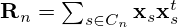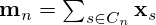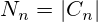These three measures help calculate the mean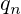and covariance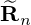. Note that the subindex here is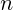- this is the tree node we're looking at. So each node in the tree has these statistical measures calculated for them.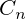refers to all pixels that belong to node.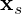refers to the RGB value at a specific pixel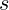.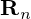andis a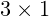matrix.

Using the second and third equations above, we can calculate the mean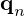of pixels belonging to a class: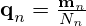Using this mean and the first equation from earlier, we can now calculate the covarianceof class.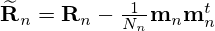These may seem daunting but they're just mathematical formulae designed to encode mean and covariance. Just remember this and not the actual mathematical formulae.

## Where do we split?

This section is key to this tutorial.

We'll use the covariance matrix for each class to figure out which class to split. The covariance matrix encodes how colors vary across the entire image. You can imagine when there's a colorful image and only one node in the tree (each pixel belongs to the same class), the covariance matrix would be crazy. As the splits happen, the covariance matrices (one for each class) would encode consecutively lower variance.

Using the covariance matrix, we can calculate a plane that separates the RGB values into two distinct parts - thus creating the split. Here's what it looks like in 2D:

Okay, so we know how to calculate the covariance and mean for each class - but how do we use them? This is where (the magical) eigenvectors show up. Eigenvectors define axes where the variance is the maximum.Eigenvectors in 2D. The eigenvectors don't need to be perpendicular as we're used to in standard coordinate spaces. Length shows the magnitude.

Here, the eigenvectors are the yellow and blue tinted arrows. They're not perpendicular and are not aligned with the coordinate axes (the black X-Y axes). Note that the yellow eigenvector encodes information that "there is maximum change along this axis". The blue eigenvector encodes the information that "there is some change along this axis - but it's not the maximum".

The above example is in two dimensions but you should be able to extend it to three dimensions (a bunch of 3D vectors in 3D space). It makes sense to somehow split the tree using the largest eigenvector.

Our datapoints are RGB values. The greater the variance along a particular axis, the greater the chances that there are actually two or more colors along that axis. In practice, this is represented by two quantities - eigenvectors and eigenvalues. Usually, eigenvectors are normalized to have unit length. Eigenvalues represent the magnitude or length of the axes.

We split the tree nodes based on the eigenvector with the largest eigenvalue. This split can contain more than one colors which we'll (hopefully) discover in subsequent splits.

## How do we split?

So now we know where do split - the eigenvalue with the largest eigenvector. Let's take a look at how to split a tree node. We'll need some mathematical notation on this one. Let's say we're splitting tree node. The variablerepresents all pixels belonging to this node. Let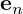represent the eigenvector with the maximum eigenvalue. Then we define new classes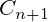and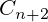using these: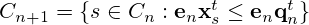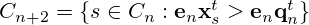This condition splits the pixels belonging to nodeinto two disjoint nodes. One way to think about this is that the eigenvector defines a normal in 3D space. Using this normal, we can define a 3D plane that splits the high variance class into two parts.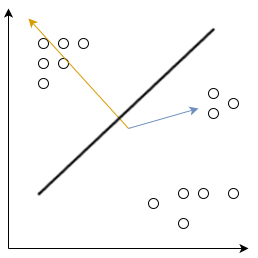First split with these eigenvector. In the next step, we calculate covariances for each class and their corresponding eigenvectors. Extrapolate this example to 3D to imagine how it'll work for our RGB data.

The normal of the 3D plane is the eigenvector. The thick line in the center is defined by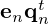. The actual datapoints being split are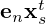whereis the RGB value at pixel.

## Onwards to implementation

Let's head on to the implementation now. I'll be using OpenCV and C++ (as always). OpenCV already comes with a bunch of useful functions (specially the `cv::eigen` method). Hopefully you enjoyed this tutorial!

### References

1. M Orchard and C Bouman, Color Quantization of Images, Transactions on Signal Processing, 1991

## More in the series

This tutorial is part of a series called Finding dominant colors in an image:

#### Related posts

Utkarsh Sinha created AI Shack in 2010 and has since been working on computer vision and related fields. He is currently at Microsoft working on computer vision.